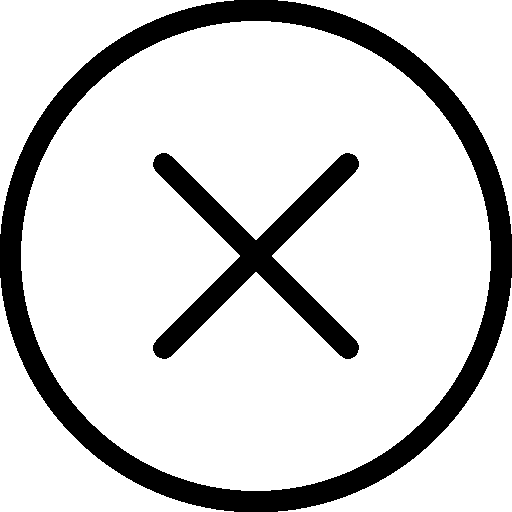• 写文章
• 提问

# 如何实现python字典循环添加元素？```dic_a = {} # 空字典

type(dic_a)
>>> dict

len(dic_a)
>>> 0```

```# 添加第一个元素
dic_a['A'] = 'Apple'

print (dic_a)
>>> {'A': 'Apple'}

# 添加第二个元素
dic_a['B'] = 'Ball'

print (dic_a)
>>> {'A': 'Apple', 'B': 'Ball'}```

```dic_a['a'] = 'apple'

print (dic_a)
>>> {'A': 'Apple', 'B': 'Ball', 'a': 'apple'}```